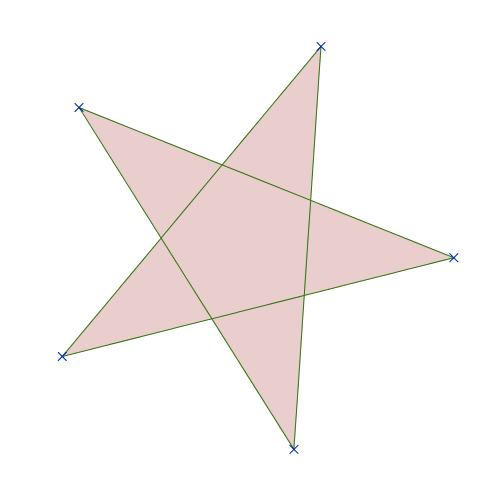Basic Mathematics

# Basic Mathematics Warmup: Level 2 Challenges

$\Large{2^{3^2}}= \, ?$Consider a regular 5 pointed star (pentagram). What is the sum of the internal angles (in degrees) of the 5 points?

$\Large \color{#3D99F6}{71^{70}} \quad \text{or} \quad \color{#D61F06}{ 70^{71} }$

Which one of the two numbers above is greater?

Which of the following triangles has a larger area:

• triangle A with side lengths $13, 13, 10$, or
• triangle B with side lengths $13, 13, 24\, ?$

$\large \color{#624F41}5^{55} + \color{#624F41}5^{55} + \color{#624F41}5^{55} + \color{#624F41}5^{55} +\color{#624F41} 5^{55} = \, ?$

×